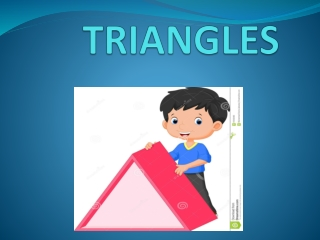DownloadDownload PresentationTriangle

# Triangle

Download Presentation## Triangle

- - - - - - - - - - - - - - - - - - - - - - - - - - - E N D - - - - - - - - - - - - - - - - - - - - - - - - - - -
##### Presentation Transcript

1. TRIANGLES

2. TRIANGLES • DEFINITION • TYPES • PROPERTIES • SECONDARYPART • CONGRUENCY

3. DEFINITION • A triangle is a polygon made up of three line segments. It is denoted by the Greek letter Δ (delta). Thus triangle PQR can be represented as ΔPQR. Every triangle has three sides, three vertices and three angles.

4. TYPES OF TRIANGLES • On Basis of Length of Sides, there are 3 types ofTriangles • Equilateral Triangle • Scalene Triangle • IsoscelesTriangle • On Basis of Angles, there are 3 types oftriangles • Acute AngledTriangle • Obtuse AngledTriangle • Right AngledTriangle

5. EQUILATERAL TRIANGLE A triangle in which all three sides are equal is called an equilateral triangle. It’s all angles are equal i.e. 60 degree. SCALENE TRIANGLE A triangle whose all three sides are unequal is called a scalene triangle.

6. ISOSCELES TRIANGLE A triangle that has two equal sides is called an isosceles triangle. The angles opposite to the equal sides are called the base angles and are also equal in size.

7. ACUTE-ANGLED TRIANGLE A triangle in which all the angles are acute angles, that is, all angles are less than 90°. OBTUSE-ANGLED TRIANGLE A triangle in which one of the angles is obtuse, that is, greater than 90°.

8. RIGHT-ANGLED TRIANGLE A triangle in which one of the angles is a right angle, that is, equal to 90°. • The other two angles of a right- angle triangle are acute angles. • The side opposite to the right angle is the largest side of the triangle and is called the hypotenuse.

9. PR0PERTIES OF A TRIANGLE • The sum of all internal angles of a triangle is always equal to 180°. This is called the angle sum property of a triangle. • The sum of the lengths of any two sides of a triangle is greater than the length of the third side. This is called triangle inequality property. • Any exterior angle of the triangle is equal to the sum of its interior opposite angles. This is called the exterior angle property of a triangle. • In a right-angled triangle, the sum of squares of the perpendicular sides is equal to the square of the hypotenuse, this is known as pythagoras’theorem

10. SECONDARY PARTS OF A TRIANGLE

11. MEDIAN OF A TRIANGLE Median is the line segment joining a vertex to the mid-point of its opposite side. ALTITUDE OF A TRIANGLE The perpendicular drawn from a vertex to its opposite side of a triangle is called an altitude.

12. PERPENDICULAR BISECTOR A perpendicular bisector can be defined as a line segment which intersects another line perpendicul- arly and divide it into two equal parts. ANGLE BISECTOR An angle bisector that divides an angle into two equal parts.

13. CONGRUENCE OF TRIANGLES

14. DEFINITION If two triangles are of the same shape and size, the triangles are said to be congruent to each other. When two triangles are congruent, the corresponding sides, angles and vertices of the two triangles will be equal. IN FIGURE, Δ ABC ≅ Δ PQR

15. CRITERIA FOR CONGRUENCE OF TRIANGLES • SAS– Side-Angle-Side • ASA – Angle-Side-Angle • AAS– Angle-Angle-Side • SSS– Side-Side-Side • RHS– Right angle-Hypotenuse-Side

16. SAS Congruence Criterion Two triangles are congruent if two sides and the included angle of one triangle are equal to the two sides and the included angle of the other triangle.

17. ASA Congruence Criterion Two triangles are congruent if two angles and the included side of one triangle are equal to two angles and the included side of the other triangle.

18. AAS Congruence Criterion Two triangles are congruent if two angles and a non-included side of a triangle are equal to the corresponding two angles and side of another triangle.

19. SSS Congruence Criterion Two triangles are congruent if three sides of one triangle are equal to the three sides of another triangle.

20. RHS Congruence Criterion Two triangles are congruent if the hypotenuse and one side of a right triangle are equal to the hypotenuse and the corresponding side of another triangle.

21. Triangles around us Triangle plays an integral role in our life from higher educational purpose to household article. For ex-Pizza and Sandwich slices, Truss bridges and pyramids

22. THANK YOU